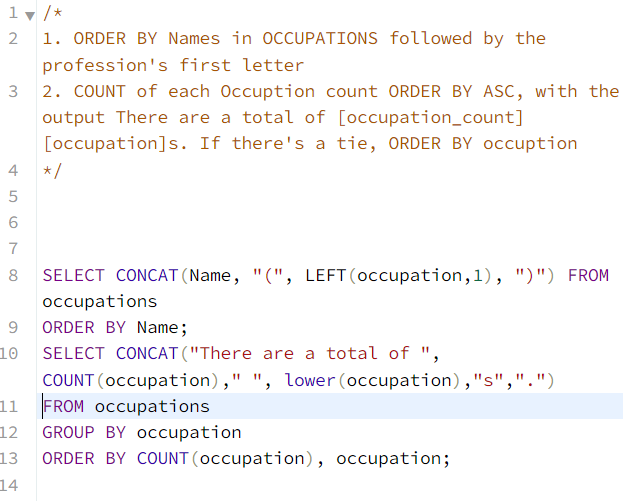Publish Date - December 15th, 2022

|

A great SQL problem, that needs a high level of attention to detail. This problem is not as technically complicated as some of the previous problems like Draw the Triangle 1 or Draw the Triangle 2. The use of CONCAT is advised for this challenge, amongst other functions because of the required output suggested by the author.

## The problem

Generate the following two result sets:

1. Query an alphabetically ordered list of all names in OCCUPATIONS, immediately followed by the first letter of each profession as a parenthetical (i.e.: enclosed in parentheses). For example: `AnActorName(A)``ADoctorName(D)``AProfessorName(P)`, and `ASingerName(S)`.
2. Query the number of ocurrences of each occupation in OCCUPATIONS. Sort the occurrences in ascending order, and output them in the following format:
``There are a total of [occupation_count] [occupation]s. where [occupation_count] is the number of occurrences of an occupation in OCCUPATIONS and [occupation] is the lowercase occupation name. If more than one Occupation has the same [occupation_count], they should be ordered alphabetically.``

Note: There will be at least two entries in the table for each type of occupation.

Input Format

The OCCUPATIONS table is described as follows:

Occupation will only contain one of the following values: DoctorProfessorSinger or Actor.

Sample Input

An OCCUPATIONS table that contains the following records:

Sample output

``````Ashely(P)
Christeen(P)
Jane(A)
Jenny(D)
Julia(A)
Ketty(P)
Maria(A)
Meera(S)
Priya(S)
Samantha(D)
There are a total of 2 doctors.
There are a total of 2 singers.
There are a total of 3 actors.
There are a total of 3 professors.``````

Explanation

The results of the first query are formatted to the problem description’s specifications.
The results of the second query are ascendingly ordered first by number of names corresponding to each profession (2 <= 2 <= 3 <= 3), and then alphabetically by profession ( doctor <= singer, actor <= professor).

## The solution

Here’s the output that you’re looking for!

``````Aamina(D)
Ashley(P)
Belvet(P)
Britney(P)
Christeen(S)
Eve(A)
Jane(S)
Jennifer(A)
Jenny(S)
Julia(D)
Ketty(A)
Kristeen(S)
Maria(P)
Meera(P)
Naomi(P)
Priya(D)
Priyanka(P)
Samantha(A)
There are a total of 3 doctors.
There are a total of 4 actors.
There are a total of 4 singers.
There are a total of 7 professors.``````

• The order in which the names appear
• brackets on the actual professions
• Spacing in the final 4 lines of output
• pluralization and punctuation of the queries in the last 4 outputs

### Here’s my query

``````/*
1. ORDER BY Names in OCCUPATIONS followed by the profession's first letter
2. COUNT of each Occuption count ORDER BY ASC, with the output There are a total of [occupation_count] [occupation]s. If there's a tie, ORDER BY occuption
*/

SELECT CONCAT(Name, "(", LEFT(occupation,1), ")") FROM occupations
ORDER BY Name;
SELECT CONCAT("There are a total of ", COUNT(occupation)," ", lower(occupation),"s",".")
FROM occupations
GROUP BY occupation
ORDER BY COUNT(occupation), occupation;
``````

As always I start by writing sudo code so that you can convert that more easily into actual code. Now, CONCAT is the most important portion of this answer because it will help you string all of your content together. Since this problem asks for two queries, I broke it up into two queries (woe is me because I thought I’d have to UNION the two queries together originally).

#### Section One:

``````SELECT CONCAT(Name, "(", LEFT(occupation,1), ")") FROM occupations
ORDER BY Name;

/* This is the first half of the query, where you will pull the name - the first letter of every record in Occupation with respect to that name in bracket. REMEMBER, to order by Name so that you end up getting the alphabetical output of names (as requested in the problem) */``````

If you just query this above, you’ll get this!

``````Aamina(D)
Ashley(P)
Belvet(P)
Britney(P)
Christeen(S)
Eve(A)
Jane(S)
Jennifer(A)
Jenny(S)
Julia(D)
Ketty(A)
Kristeen(S)
Maria(P)
Meera(P)
Naomi(P)
Priya(D)
Priyanka(P)
Samantha(A)``````

Pretty easy right?

#### Section Two:

``````SELECT CONCAT("There are a total of ", COUNT(occupation)," ", LOWER(occupation),"s",".")
FROM occupations
GROUP BY occupation
ORDER BY COUNT(occupation), occupation;

/* Note the CONCAT with the lower case on the occupation, the spacing, the "s" and the ".". This is so that you can get that nice string that's requested in the end. (EX: There are a total of 3 doctors.). From there, you'll need to GROUP BY because you're aggregating and then ORDER BY twice due to the requirements in the question */``````

## Conclusion

Great question overall that had be overthinking once again. After all of my attempts at trying to SET a variable for and do a self / sub-query, I realized I could just string concatenate.. Occam’s Razor – sometimes the best approach is the simplest!

Check out some of my guides for Udemy, where I learned to code!